#### All Pages

Assignment Help: >> Random Search Optimisation Techniques - Objectives of Loading Problems

In any type of manufacturing system, there are different objectives associated along with machine loading problems, as:

(a) Minimization of work in process or WIP inventory

(c) Maximization of system throughput

(d) Minimization of Make span

(e) Minimization of system unbalance

(f) Maximization of profit

In the present chapter, two subjective namely minimization of system unbalance and maximization of throughput are taken, where system disturb or unbalance is the net sum of remaining time on all machines after allocation of each operation of the task and throughput is the total of the batch size of the chosen jobs. Minimum value of system disturbs or unbalance can be zero this is worth to mention that overloading of the machines is permitted and the maximum value of the throughput is while each job get chosen. Mathematically, the afore-mentioned objective can be written like:

System unbalance or US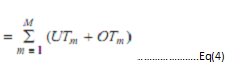In the afore-mentioned equation, UTm denotes the underutilized time upon machine m and OTm is the over-utilized time on machine m. The index of machine is m.

Throughput or TH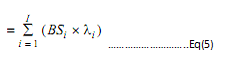BSi is the batch size of job i and λ is the decision variable equivalents 1, if job is chosen for processing, or else 0. Because, in the afore-mentioned objectives, system disturb or unbalance is to be minimum and throughput is to be maximum, hence, an overall weighted objective function is formed via transforming the minimization of system disturb or unbalance value into the maximization of system balance value, described as: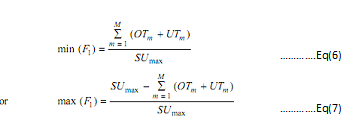Here, SUmax is the maximum possible value of system unbalance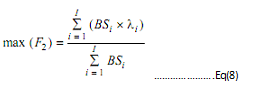hence, the overall objective function will be,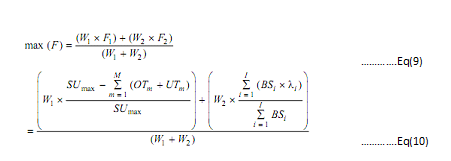A step-wise process to calculate the afore-mentioned objectives is described as:

Step 1

Input the total number of machines mmax, here m is the index for machines,

m = 1, 2, . . . , mmax. For m = 1 to mmax, input the ATMM and ATSM, where, ATMM   = Available machining time on machine m, ATSM = available tools slots on machine m.

Step 2

Input the job sequence having jmax jobs arranged at kmax positions, here j is the index for task, j = 1 to jmax, and k is the index for the location of the part type in the manner, k = 1 to kmax.

Step 3

Initialize K = 1, and O = 1.

Step 4

As per to the input sequence, for the job j at kth position, If the task contains necessary operation,

Then for the necessary operation O, find out ETMjom, ETSjom, ATMm, ATSm, here, ETMjom is the important time requirement of job j for operation O on machine m, ETMjom is the important tool slot requirement of job j for operation O upon machine m.

Step 5

If ATSm ≥ ETSjom,

Then go to Step 6,

Else, reject the job j (tool slot constraints) and increase index k by 1 (i.e. k = k + 1)

and goto Step 4.

Step 6

If ATMm ≥ ETMjom,

Then assign the necessary operation o of the job on machine m. find out RTMm, RTSm, where, RTMm is the keep time on the machine m and RTSm is the saying tool slot on machine m after the allocation. At this time, update the available time and tool slot, consequent to machine m, that is (ATMm = RTMm and ATSm = RTSm).

Else determine SUjom, where, SUjom is the system unbalance after allocation of Oth

operation of job j on machine m.

If (SUjom < 0),

Then refuse the job j (negative system unbalance), now increase k by 1 (k = k + 1), Go to Step 4.

Else allocate the necessary operation O of job J upon machine m. find out RTMm, and RTSm and update the obtainable time and tool slot to the corresponding that is

ATMm = RTMm and ATMm = RTMm.

Step 7

If O < Omax

Then increase O by 1 (that is O = O + 1) and go to Step 6.

Else go to Step 8.

Step 8

Find out OTMJOM and OTSJOM for the entire optional operations o (that is set of operations that have more than one machine as an option to process). O = 1 to Omax, OTMJOM is the machining time of the optional operation O of the job j on machine m. and OTSJOM is the machining time of the optional operation O of the job j upon machine m.

Step 9

Initialize O = 1;

Step 10

If (ATMm ≥ OTMjom), Then go to Step 11, Else evaluate SU,

If (SU > 0)

Then go to Step 11,

Else reject the job (negative system unbalance), and set k = k + 1, and go to Step 4.

Step 11

If (ATSm ≥ OTSjom)

Then assign the optional operation O of job j on machine m. find out RTMm, RTSm after allocation. Set (ATMm = RTMm, and ATSm = RTSm) go to

Step 12.

Else reject the job j (tool slot constraint), set k = k + 1.

Step 12

If (O < Omax),

Then set O = O + 1, go to Step 10,

Else go to Step 13.

Step 13

If (k < kmax)

Then go to Step 2,

Else go to Step 14.

Step 14

Find out system unbalance SU, Throughput TH, set of assigned jobs and set of jobs rejected due to tool slot constraint (JRTSC) and negative system unbalance (JRNSU).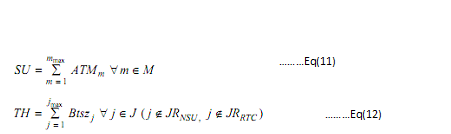Step 15

As per to objective function, evaluate the fitness value.

Table no.1: Initial Available Data

 Machine No. Available Time Available Tool Slot 1 480 5 2 480 5 3 480 5 4 480 5